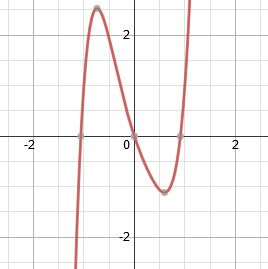# Terminology of Polynomial Functions

An error occurred trying to load this video.

Try refreshing the page, or contact customer support.

Coming up next: How to Evaluate a Polynomial in Function Notation

### You're on a roll. Keep up the good work!

Replay
Your next lesson will play in 10 seconds
• 1:19 Coefficients
• 1:58 Terms
• 3:37 Degree
• 4:19 Putting It Together
• 5:04 Lesson Summary

Want to watch this again later?

Timeline
Autoplay
Autoplay
Speed

#### Recommended Lessons and Courses for You

Lesson Transcript
Instructor: Yuanxin (Amy) Yang Alcocer

Amy has a master's degree in secondary education and has taught math at a public charter high school.

After watching this video lesson, you will be able to understand and use the words associated with polynomial functions like a pro. You will see words, such as leading coefficient, and you will immediately know what it is referring to.

## Polynomial Functions

This video lesson is all about the keywords associated with polynomial functions. A polynomial is made up of several combinations of constants, variables, and exponents. Recall that a constant is simply a number, a variable is a letter, and exponents are the powers that the variable is raised to. When you graph a polynomial, you will get a line that looks like a roller coaster.This graph is showing the polynomial function f(x) = 4x^5 - x^4 - x^3 + 2x^2 - 3x. See how it looks like a roller coaster? I personally wouldn't get on this roller coaster. It looks a bit scary to me. But I'll talk about the polynomial function any day.

This polynomial function is written in standard form because the exponents linked to our variables are written from large to small. Our first exponent is 5, followed by a 4, then the 3, then the 2, then a 1.

Why should you be interested in learning about keywords associated with polynomials? Well, because you will come across these terms more and more often the more you learn about polynomials. Also, the more math you do, the more polynomials you will come across. You will see polynomials in real life, and if you understand these terms, you will be in a better position to talk about them. Are you ready to dig in?

## Coefficients

If you look at the polynomial function we just graphed, you will see numbers in front of the variables. These numbers are called our coefficients. If we don't see a number, then we have an invisible 1. So our coefficients going from left to right are 4, -1, -1, 2, and -3. Because our polynomial is written in standard form, the very first coefficient is our leading coefficient. It is always the coefficient linked to the variable with the highest exponent. For our polynomial, the leading coefficient is 4.

## Terms

Next, we have our terms. The terms are products of constants and variables with their exponents. For polynomials, the exponents of our terms cannot be negative nor can they be fractions; the exponents must be positive whole numbers. For example, 4x and 2x^2 are both valid terms. But what if you have 4x^-1 or x^(1/2)? Are these valid terms for polynomials? No, they are not.

Why is this? The first isn't valid because it has a negative exponent. The second isn't valid because it has a fractional exponent. Look back at our polynomial that we graphed. This polynomial has a total of five terms. We have 4x^5, -x^4, -x^3, 2x^2, and -3x. Do you see how all our terms have exponents that are positive whole numbers? Do you also see how our polynomial is made up of separate terms separated by either addition or subtraction? If we have subtraction, then it means the term is negative. If we have addition, then our term is positive. We can also have terms that are just variables or even just numbers. If it is just a number, we sometimes refer to it as a constant term.

To unlock this lesson you must be a Study.com Member.

### Register to view this lesson

Are you a student or a teacher?

### Unlock Your Education

#### See for yourself why 30 million people use Study.com

##### Become a Study.com member and start learning now.
Back
What teachers are saying about Study.com

### Earning College Credit

Did you know… We have over 160 college courses that prepare you to earn credit by exam that is accepted by over 1,500 colleges and universities. You can test out of the first two years of college and save thousands off your degree. Anyone can earn credit-by-exam regardless of age or education level.